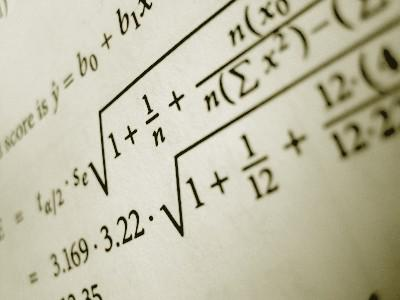COURSE DESCRIPTIONSection 1: Statistics
An introduction to statistics including an overview and data classification.
Descriptive statistics including frequency distributions, measures of central tendency, variation and position.
Normal probability distributions including standard normal distributions, the central limit theorem, and normal approximations to the binomial distribution.
Confidence intervals for the mean, population proportion and standard deviation.
Section 2: Probability
Concepts of probability, including conditional probability, probability rules and counting principles.
Discrete distributions including probability and binomial distributions.
Three examinations are given during the course. Quizzes are at the end of each topic. Use the links page to help offer further definitions and examples.

CHAPTERSCh.1: Independent and Dependent Events
Ch.2: The Next Step…Conditional Probability
Ch.3: Introduction to Discrete Random Variables
Ch. 4: Probability Distributions
Ch.5: Measures of Central Tendency
Ch.6: Shape, Center and Spread of a Normal Distribution
Ch.7: Organizing and Displaying Distributions of Data
Ch.8: Organizing and Displaying Data for Comparison